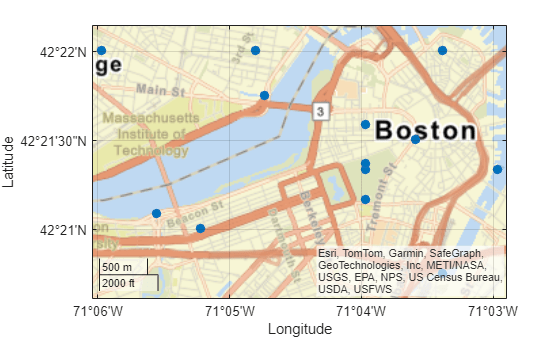# geopointshape

Point shape in geographic coordinates

## Description

A `geopointshape` object represents a point or multipoint in geographic coordinates. A multipoint is an individual point shape that contains a set of point locations.

To represent a point or multipoint in planar coordinates, use a `mappointshape` object instead.

## Creation

To create `geopointshape` objects, either:

• Import point data in geographic coordinates as a geospatial table using the `readgeotable` function, and then query the `Shape` variable of the table.

• Use the `geopointshape` function (described here).

### Syntax

``shape = geopointshape(lat,lon)``

### Description

example

````shape = geopointshape(lat,lon)` creates a `geopointshape` object or array of `geopointshape` objects with the specified latitude and longitude coordinates. The sizes of `lat`, `lon`, and the `geopointshape` object array `shape` match.```

### Input Arguments

expand all

Latitude coordinates, specified as a numeric array or a cell array of numeric arrays.

• Create a point by specifying a scalar, such as `39`.

• Create a multipoint by specifying an array within a cell, such as `{[38 -30 29]}`.

• Create an array of points by specifying an array, such as ```[38 -30 29]```.

• Create an array of points and multipoints by specifying a cell array of numeric arrays, such as `{39,[38 -30 29]}`.

Create placeholders for points with missing data by including `NaN` values. The `NaN` values in `lat` must correspond to the `NaN` values in `lon`.

The size of `lat` must match the size of `lon`. For cell arrays, the size of the array in each cell of `lat` must match the size of the array in the corresponding cell of `lon`.

Data Types: `double` | `cell`

Longitude coordinates, specified as a numeric array or a cell array of numeric arrays.

• Create a point by specifying a scalar, such as `-113`.

• Create a multipoint by specifying an array within a cell, such as `{[-66 -31 42]}`.

• Create an array of points by specifying an array, such as ```[-66 -31 42]```.

• Create an array of points and multipoints by specifying a cell array of numeric arrays, such as `{-113,[-66 -31 42]}`.

Create placeholders for points with missing data by including `NaN` values. The `NaN` values in `lat` must correspond to the `NaN` values in `lon`.

The size of `lat` must match the size of `lon`. For cell arrays, the size of the array in each cell of `lat` must match the size of the array in the corresponding cell of `lon`.

## Properties

expand all

Number of points, returned as an array of nonnegative integers.

For a `geopointshape` scalar, the value of `NumPoints` is `1` when the `geopointshape` object represents a single point and more than `1` when the object represents a multipoint.

For a `geopointshape` array, the size of `NumPoints` matches the size of the array.

Data Types: `double`

Latitude coordinates, specified as an array.

For a `geopointshape` scalar, the size of `Latitude` matches the value of `NumPoints`.

For a `geopointshape` array, the size of `Latitude` matches the size of `NumPoints`. If the array contains `geopointshape` objects with multipoints, then accessing the `Latitude` property of the array is not supported. Instead, access the `Latitude` property of individual objects within the array.

This property is read-only for arrays when any element of `NumPoints` is greater than `1`.

`Latitude` and `Longitude` must be the same size.

Data Types: `double`

Longitude coordinates, specified as an array.

For a `geopointshape` scalar, the size of `Longitude` matches the value of `NumPoints`.

For a `geopointshape` array, the size of `Longitude` matches the size of `NumPoints`. If the array contains `geopointshape` objects with multipoints, then accessing the `Longitude` property of the array is not supported. Instead, access the `Longitude` property of individual elements within the array.

This property is read-only for arrays when any element of `NumPoints` is greater than `1`.

`Latitude` and `Longitude` must be the same size.

Data Types: `double`

Geometric type, returned as `"point"`.

Data Types: `string`

Coordinate system type, returned as `"geographic"`.

Data Types: `string`

Geographic coordinate reference system (CRS), specified as a `geocrs` object. A geographic CRS consists of a datum (including its ellipsoid), prime meridian, and angular unit of measurement.

## Object Functions

 `geoplot` Plot points, lines, and polygons in geographic axes `geoclip` Clip geographic shape to latitude-longitude limits

## Examples

collapse all

Import a GPX file containing the coordinates of locations in Boston as a geospatial table. The GPX file represents the locations using points. Get information about the points by querying the `Shape` variable of the table.

```GT = readgeotable("boston_placenames.gpx"); GT.Shape```
```ans = 13×1 geopointshape array with properties: NumPoints: [13×1 double] Latitude: [13×1 double] Longitude: [13×1 double] Geometry: "point" CoordinateSystemType: "geographic" GeographicCRS: [1×1 geocrs] ```

Display the locations on a road map by passing the geospatial table to the `geoplot` function.

```figure geoplot(GT,"o",MarkerFaceColor="#0072BD") geobasemap streets```Create an individual point as a `geopointshape` scalar. Specify the geographic CRS as the World Geodetic System of 1984, which has the EPSG code `4326`.

```point = geopointshape(39,-113); g = geocrs(4326); point.GeographicCRS = g```
```point = geopointshape with properties: NumPoints: 1 Latitude: 39 Longitude: -113 Geometry: "point" CoordinateSystemType: "geographic" GeographicCRS: [1x1 geocrs] ```

Create a multipoint as a `geopointshape` scalar.

```multipoint = geopointshape({[38 -30 29]},{[-66 -31 42]}); multipoint.GeographicCRS = g```
```multipoint = geopointshape with properties: NumPoints: 3 Latitude: [38 -30 29] Longitude: [-66 -31 42] Geometry: "point" CoordinateSystemType: "geographic" GeographicCRS: [1x1 geocrs] ```

Create three individual points as a 1-by-3 `geopointshape` vector.

```pointVector = geopointshape([38 -20 29],[-66 -31 42]); pointVector.GeographicCRS = g```
```pointVector = 1x3 geopointshape array with properties: NumPoints: [1 1 1] Latitude: [38 -20 29] Longitude: [-66 -31 42] Geometry: "point" CoordinateSystemType: "geographic" GeographicCRS: [1x1 geocrs] ```

Create one individual point and one multipoint as a 1-by-2 `geopointshape` vector.

```pointMultipoint = geopointshape({39,[38 -30 29]},{-113,[-66 -31 42]}); pointMultipoint.GeographicCRS = g```
```pointMultipoint = 1x2 geopointshape array with properties: NumPoints: [1 3] Geometry: "point" CoordinateSystemType: "geographic" GeographicCRS: [1x1 geocrs] ```

## Version History

Introduced in R2021b

expand all

Behavior changed in R2022a